Click in-app to access the full platform documentation for your version of DataRobot.

# Data tab¶

Once DataRobot displays features on the Data page, you can click a feature name to view its details and also (in some cases) modify its type. The options available are dependent on variable type:

Option Description Variable Type
Tabs
Histogram Buckets numeric feature values into equal-sized ranges to show a rough distribution of the variable. numeric, summarized categorical, multicategorical
Frequent Values Plots the counts of each individual value for the most frequent values of a feature. If there are more than 10 categories, DataRobot displays values that account for 95% of the data; the remaining 5% of values are bucketed into a single "All Other" category. numeric, categorical, text, boolean
Table Provides a table of feature values and their occurrence counts. Note that if the value displayed contains a leading space, DataRobot includes a tag, leading space, to indicate as much. This is to help clarify why a particular value may show twice in the histogram (for example, 36 months and 36 months are both represented). numeric, categorical, text, boolean, summarized categorical, multilabel
Illustration Shows how summarized categorical data—features that host a collection of categories—is represented as a feature. See also the summarized categorical tab differences for information on Overview and Histogram. summarized categorical
Category Cloud After EDA2 completes, displays the keys most relevant to their corresponding feature in Word Cloud format. This is the same Word Cloud that is available from the Category Cloud on the Insights page. From the Data page you can more easily compare clouds across features; on the Insights page you can compare word clouds for a project's categorically-based models. summarized categorical
Feature Statistics Reports overall multilabel dataset characteristics, as well as pairwise statistics for pairs of labels and the occurrence percentage of each label in the dataset. multilabel
Over Time (time-aware only) Identifies trends and potential gaps in data by displaying, for both the original modeling data and the derived data, how a feature changes over the primary date/time feature. numeric, categorical, text, boolean
Feature Lineage (time series) or (Feature Discovery) Provides a visual description of how a derived feature was created. numeric, categorical, text, boolean
Actions
Var Type Transform Provides a dialog to modify the variable type. (Not shown if the variable type for this feature was previously transformed.) numeric, categorical, text
Transformation Shows details for a selected transformed feature and a comparison of the transformed feature with the parent feature. (Applies to transformed features only.) numeric, boolean

Note

The values and displays for a feature may differ between EDA1 and EDA2. For EDA1, the charts represent data straight from the dataset. After you have selected a target and built models, the data calculations may have fewer rows due to, for example, holdout or missing values. Additionally, after EDA2 DataRobot displays average target values which are not yet calculated for EDA1.

## Histogram chart¶

The Histogram chart is the default display for numeric features. It represents a frequency distribution—the target observation (left Y-axis) plotted against the frequency of the value (X-axis). When you expand a numeric feature from the Data page, DataRobot displays its histogram. The display differs depending on whether a data quality issue was found. With no issues: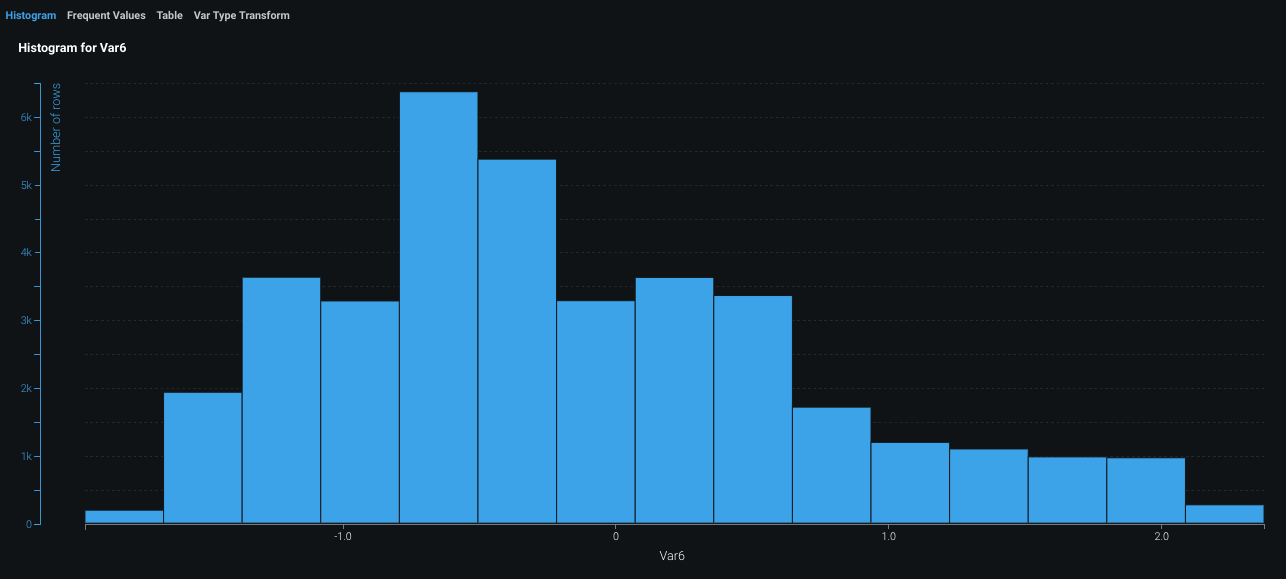With data quality issues: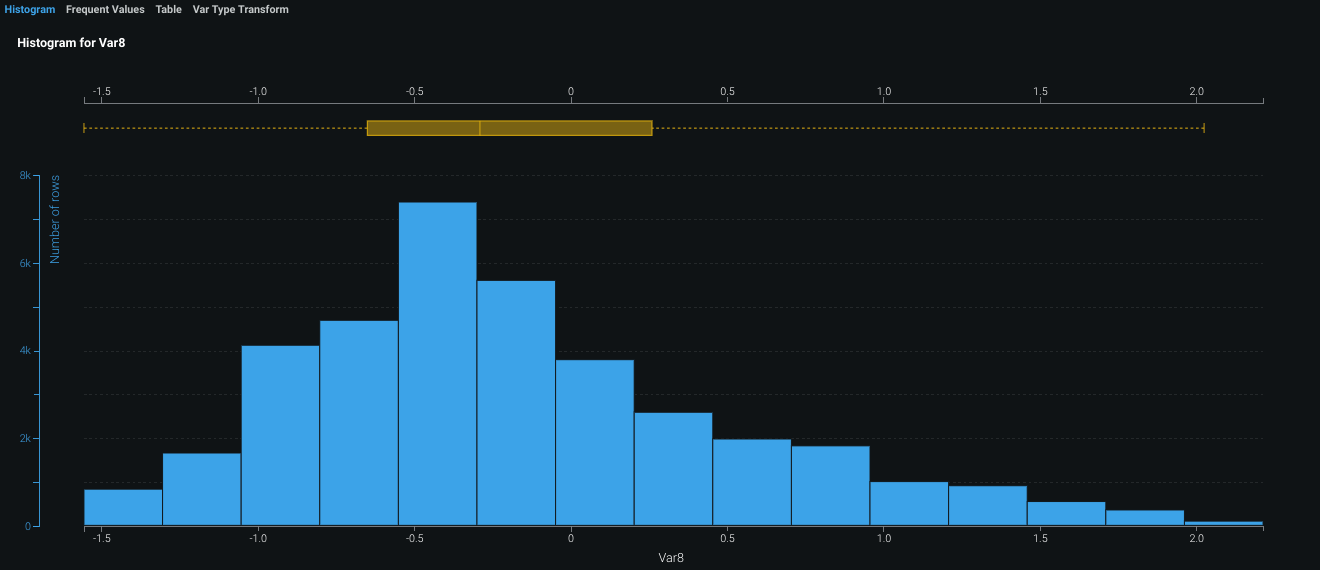After EDA2 completes, the histogram also displays an average target value overlay.

### Change the distribution and display¶

DataRobot breaks the data into several bins; the size of the bin depends on the number of rows in your dataset. You can change the number of bins to change the distribution range. The bin options depend largely on the number of unique values in the dataset. To change the distribution range use the dropdown:For classification projects, you can also (after EDA2) change the basis of the display to fill bins based on the number of rows or percentage of target value. The displays of the histogram and average target value overlay also change to match your selection.

### Display summaries¶

To see the details of a selected bin, hover over the bin until a popup displays:• The bin range (1) is located on the X-axis.
• The number of rows (2) is located on the left Y-axis.
• The average target value (3) is located on the right Y-axis.

### Calculate outliers¶

Outliers, the observation points at the far ends of the sample mean, may be the result of data variability. They can also represent data error, in which case you may want to exclude them from the histogram. Outlier detection—run as part of EDA1 using a combination of heuristics—is strictly a histogram visualization tool and does not influence the modeling process.

Outliers are generally calculated as a collection of two ranges:

• `p25` represents the values in the first quartile of a data distribution.
• `p75` represents the values in the third quartile of a data distribution.
• `IQR` is the Interquartile Range, equal to the difference of the first quartile subtracted from the third quartile: `IQR = p75-p25`.

The ranges are then calculated as the first quartile minus IQR (`p25-IQR`) and the third quartile plus IQR (`p75+IQR`). Note that this is a general overview of outlier calculation. Additional calculations are required depending on how these ranges compare to the minimal and maximal values of the data distribution. There are also additional heuristics used for corner cases that cover how DataRobot calculates IQR and the final values of the outlier threshold.

Check the Show outliers box and to initiate a calculation identifying the rows containing outliers. DataRobot then re-displays the histogram with outliers included: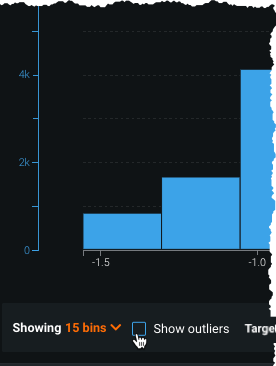Check and uncheck the box to switch the histogram display between off (excluding) and on (including) outliers:

Note that DataRobot reshuffles the bin values based on the display. With outliers excluded, there are more rows and each contains a smaller number of rows. When toggled on, each bin contains a greater number of rows because the bin has expanded its range of values.

The bin selection dropdown works as usual, regardless of the outlier display setting.

## Frequent Values chart¶

The Frequent Values chart is the default display for categorical, text, and boolean features, although it is also available to other feature types. The display is dependent on the results of the data quality check. With no data quality issues: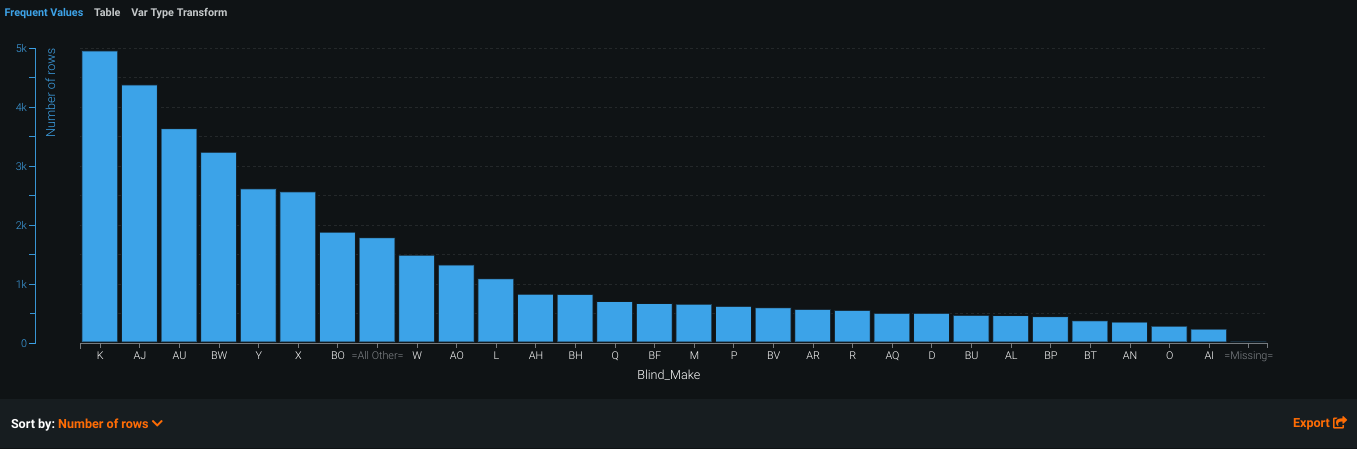In many cases, you can change the display using the Sort by dropdown. By default, DataRobot sorts by frequency (Number of rows), from highest to lowest. You can also sort by <feature_name>, which displays either alphabetically or, in the case of numerics, from low to high. The Export link allows you to download an image of the Frequent Values chart as a PNG file.

After EDA2 completes, the Frequent Values chart also displays an average target value overlay.

## Summarized categorical features¶

The summarized categorical variable type is used for features that host a collection of categories (for example, the count of a product by category or department). If your original dataset does not have features of this type, DataRobot creates them (where appropriate as described below) as part of EDA2. The summarized categorical variable type offers unique feature details in its Overview, Histogram, Category Cloud, and Table tabs.

Note

You cannot use summarized categorical features as your target for modeling.

### Required dataset formatting¶

For features to be detected as the summarized categorical variable type (shown in the Var Type column on the Data tab), the column in your dataset must be a valid JSON-formatted dictionary:

`"Key1": Value1, "Key2": Value2, "Key3": Value3, ...`

• `"Key":` must be a string.
• `Value` must be numeric (an integer or floating point value) and greater than 0.
• Each key requires a corresponding value. If there is no value for a given key, the data will not be usable.
• The column must be JSON-serializable.

The following is an example of a valid summarized categorical column:

`{“Book1”: 100, “Book2”: 13}`

An invalid summarized categorical column can look like any of the following examples:

• `{‘Book1’: 100, ‘Book2’: 12}`

• The key is not in double inverted commas (not JSON-serializable).
• `{‘Book1’: ‘rate’,‘Book2’: ‘rate1’}`

• These values are strings instead of positive numeric values.
• `{“Book1”, “Book2”}`

• This example is not in JSON dictionary format.

### Overview tab for summarized categorical¶

The Overview tab presents the top 50 most frequent keys for your feature. Each key displays the percentage of rows that it appears in, its mean, standard deviation, median, min, and max. You can sort the keys by any of these fields. Most of this information is available for other types of features in the columns on the Data page, but for summarized categorical features each individual key has its own values for these fields.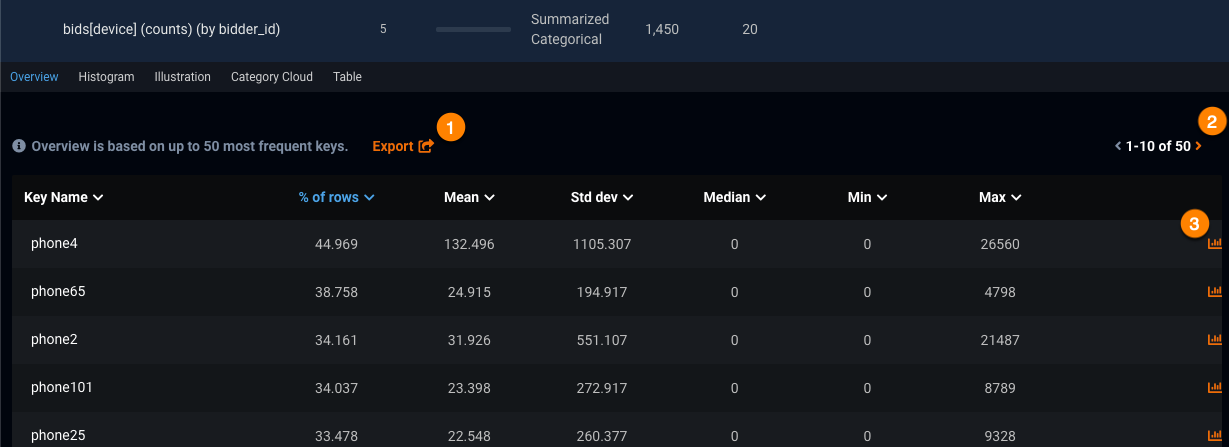From the Overview tab, you can:

• Export (1) the list of keys and their associated values as a PNG. You can choose to include the chart title in the image and edit the filename before you download it.
• Move (2) through pages of listed keys (10 keys per page).
• Access the histogram (3) for a given key.

### Histogram tab for summarized categorical¶

While most of the functionality for this tab is the same as described in the working with histograms section above, there are some differences unique to this variable type. The histograms displayed in this tab correspond to the individual labels (keys) of a feature instead of a feature itself. The list of keys can be sorted by percentage of occurrence in the dataset's rows or alphabetically.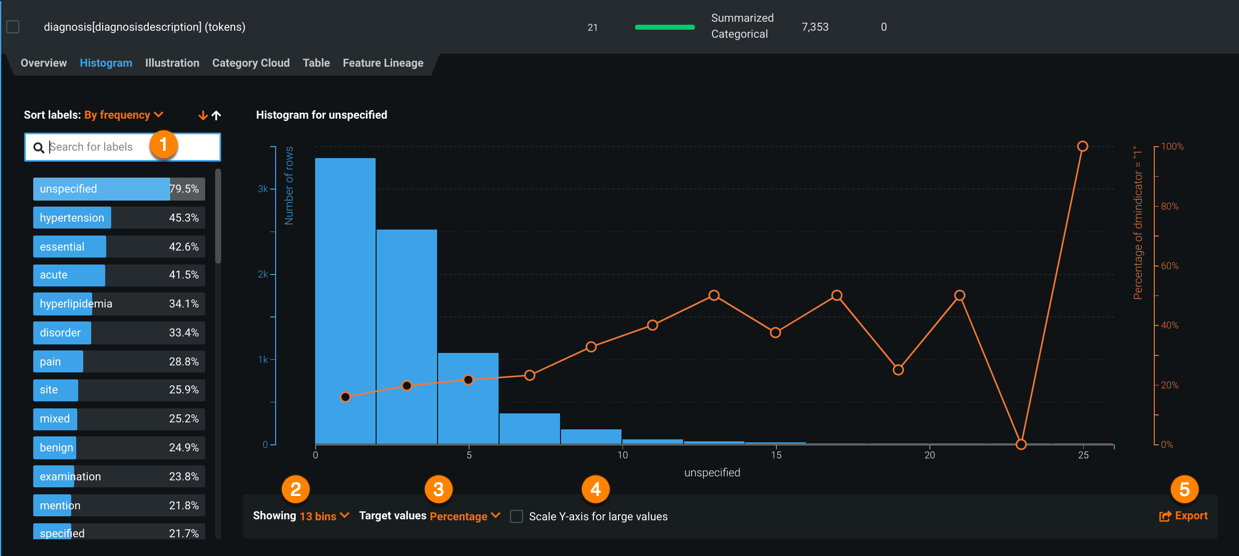DataRobot automatically filters out stopwords when calculating values for the histogram.

From the Histogram tab, you can:

• Search (1) for labels.
• Change the bin distribution (2).
• Set the basis of the target value display (3).
• Scale the Y-axis for large values (4). This will reduce the number of rows measured in the Y-axis to improve the visualization of larger values, as it is common that they are only represented in a few rows. Resizing the histogram above results in: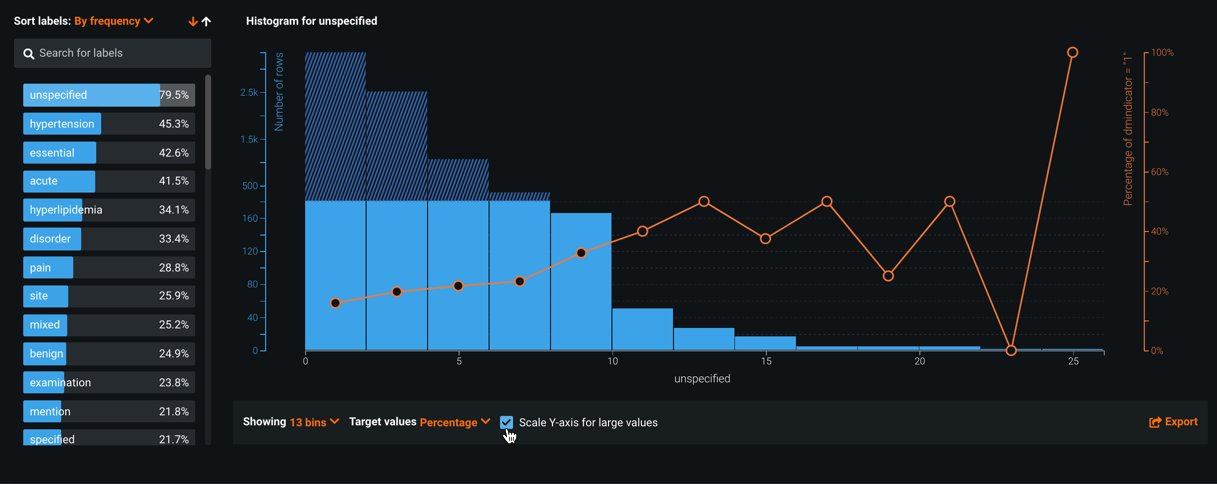By scaling the Y-axis, you can see that the greatest value measured has been greatly reduced. As a result, the number of rows across all values are more evenly represented.

## Illustration table¶

The Illustration tab shows how summarized categorical data is represented as a feature. For example, in the below image, the Values column contains five summarized categorical features displayed in JSON dictionary format (selected at random), as described above.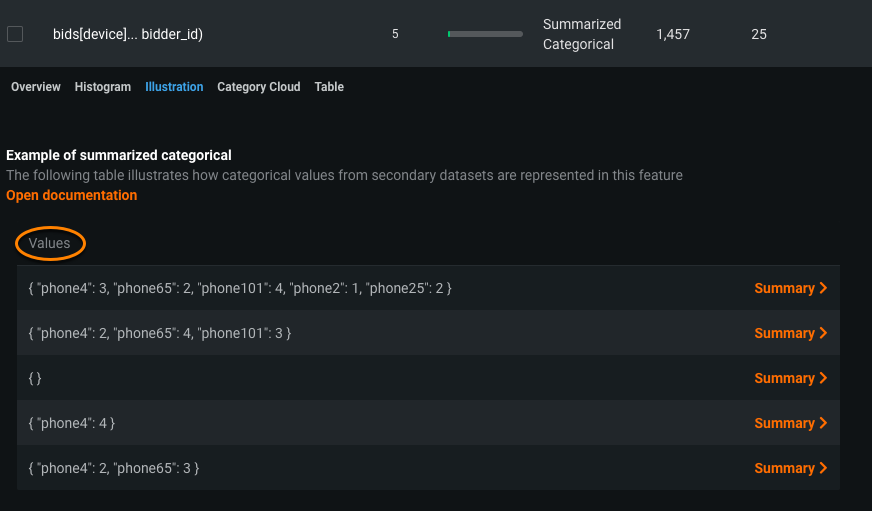Click Summary to display a box that visualizes how categorical values appeared in their initial state, prior to being engineered as summarized categorical features.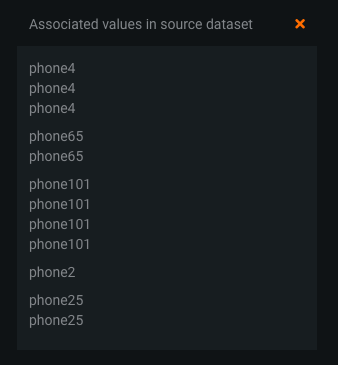## Table tab¶

The Table tab, which is the default tab for multilabel projects, displays a two-column table detailing counts for the top 50 most frequent label sets in the multicategorical feature.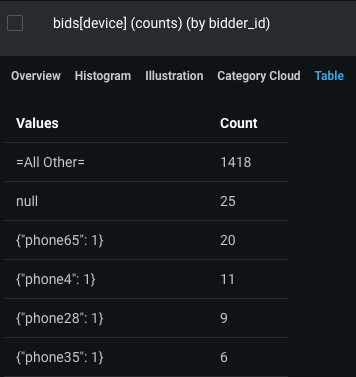The table lists each key in the Values column, and the respective key's count in the Count column.

## Average target values¶

After EDA2, DataRobot displays orange circles as graph overlays on the Histogram and Frequent Values charts. The circles indicate the average target value for a bin. (These circles are connected for numeric features and not for categorical, since the ordering of categorical variables is arbitrary and histograms display a continuous range of values.)

For example, consider the feature `num_lab_procedures`: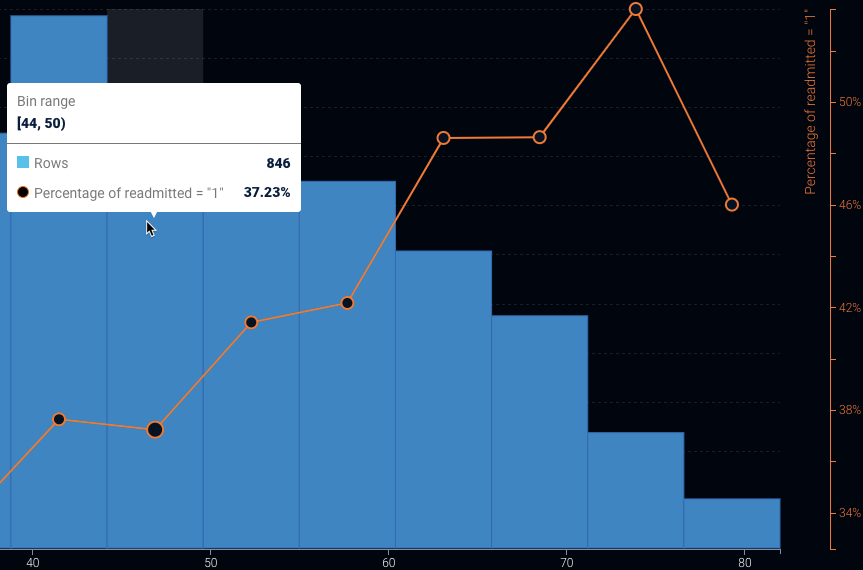In this example, there are 846 people who had between 44-49.999999 lab procedures. The average target value represented by the circle (in this case, the percent readmitted) is 37.23%. (The orange dots correspond to the right axis of the histogram.)

### How Exposure changes output¶

If you used the Exposure parameter when building models for the project, the Histogram and Frequent values tabs display the graphs adjusted to exposure. In this case: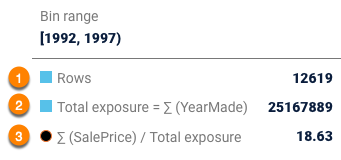• The number of rows (1) in each bin.
• The sum of exposure (2) in each bin. That is, the sum of the weights for all rows weighted by exposure.
• The sum of target value divided by the sum of the exposure (3) in a bin.

### How Weight changes output¶

If you set the Weight parameter for a project, DataRobot weights the number of rows and average target values by weight.

Updated November 17, 2021